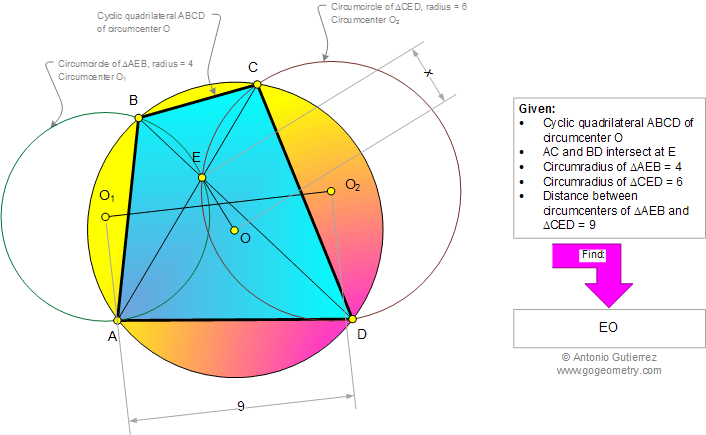# Geometry Problem 938: Cyclic Quadrilateral, Triangle, Circumcircle, Circles, Circumcenter, Circumradius, Distance, Metric Relations. Level: High School, College, Mathematics Education

< PREVIOUS PROBLEM  |  NEXT PROBLEM >

 The figure below show a cyclic quadrilateral ABCD with the circumcenter O. AC and BD intersect at E. The measures of circumradii of triangles AEB and CED are equal to 4 and 6, respectively. If the distance between the circumcenters of triangles AEB and CED is equal to 9, find EO.Home | Search | Geometry | Problems | All Problems | Open Problems | Visual Index | 10 Problems | Problems Art Gallery |  Art | 931-940 | Cyclic Quadrilateral | Triangles | Circle | Circumcircle | by Antonio Gutierrez